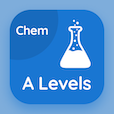Online College Courses

College Chemistry Quizzes

College Chemistry Quiz - Complete

Practice Partial Pressure Calculations quiz questions and answers, partial pressure calculations MCQ questions PDF, test 141 to study College Chemistry online course. Gases MCQ questions, partial pressure calculations Multiple Choice Questions (MCQ Quiz) for online college degrees. Partial Pressure Calculations Book PDF: rutherford model of atom, azimuthal quantum number, ionization energy periodic table, liquid crystals, partial pressure calculations test prep for 2 year online degrees.

"Sum of mole fraction of all gases is equal to" Quiz PDF: partial pressure calculations App APK with five, two, six, and unity choices for GRE prep classes. Solve gases questions and answers to improve problem solving skills for free online classes.

## Trivia Questions on Partial Pressure Calculations MCQs

MCQ: Sum of mole fraction of all gases is equal to

two
five
six
unity

MCQ: In breast cancer liquid crystals show

yellow colour
green colour
blue colour
purple colour

MCQ: The force of attraction decreases due to an increase in

stark effect
shielding effect
screening effect
Zeeman effect

MCQ: The formula for calculating electron is

2(2l+1)
(2l+1)
2(2l)
2(2l+2)

MCQ: The number of protons in the nucleus is similar to the number of

neutrons
neutrino
positrons
electrons

### More Quizzes from College Chemistry Book